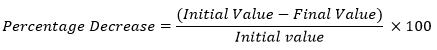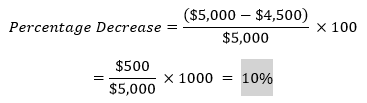# Percentage Decrease Calculator

To calculate the percentage decrease, enter the initial and final value in the input boxes and hit the calculate button using this percentage decrease calculator

Give Us Feedback

With our percentage decrease calculator, you can find the difference between an initial value and a final value in terms of percentage.

You can enter the starting and ending values in the provided spaces, and our calculator will tell you the decrease between them in the form of a percentage.

## How to Use This Percentage Decrease Calculator?

To calculate the percentage decrease between two values, follow the below steps:

1. Enter the initial and final value in the given spaces
2. Click the calculate button

You can also use our percentage increase calculator if your final value is greater than the initial value.

## What is a Percentage Decrease?

Percentage decrease can be defined as the representation of the reduction between an initial value and a final value in the form of a percentage.

## The formula for Computing Percentage Decrease

You can use this formula to manually find out the percentage decrease between an initial and final value.## Percentage Decrease Example

Here is an example to help us understand the concept of percentage decrease better.

Example:

An accountant earns \$5,000 per month. Due to poor performance, his salary is decreased by \$500. His monthly payment is now \$4,500.

To calculate the percentage decrease in the salary of the accountant, we can use the formula given above.We can say that there has been a 10% decrease in the monthly salary of the accountant.

### Math Tools